# MA.912.F.1.5Export Print
Compare key features of linear functions each represented algebraically, graphically, in tables or written descriptions.

### Clarifications

Clarification 1: Key features are limited to domain; range; intercepts; slope and end behavior.
General Information
Subject Area: Mathematics (B.E.S.T.)
Strand: Functions
Status: State Board Approved

## Benchmark Instructional Guide

• Domain
• Intercept
• Range
• Slope

### Vertical Alignment

Previous Benchmarks

Next Benchmarks

### Purpose and Instructional Strategies

In grade 8, students interpreted the slope and $y$-intercept of a linear equation in two variables. In Algebra I, students compare key features of two or more linear functions. In later courses, students will compare key features of linear and nonlinear functions.
• Instruction includes the use of various forms of linear equations. Additionally, linear functions can be represented as a table of values or graphically.
• Standard Form
Can be described by the equation $A$$x$ + $B$$y$ = $C$, where $A$, $B$ and $C$  are any rational number.
• Slope-Intercept Form
Can be described by the equation $y$ = $m$$x$ + $b$, where $m$ is the slope and $b$ is the $y$-intercept.
• Point-Slope Form
Can be described by the equation $y$ − $y$1 = $m$($x$$x$1), where ($x$1, $y$1) are a point on the line and $m$ is the slope of the line.
• Problem types include comparing linear functions presented in similar forms and in different forms, and comparing more than two linear functions.
• Instruction includes representing domain and range using words, inequality notation and set-builder notation.
• Words
If the domain is all real numbers, it can be written as “all real numbers” or “any value of $x$, such that $x$ is a real number.”
• Inequality notation
If the domain is all values of $x$ greater than 2, it can be represented as $x$ > 2.
• Set-builder notation
If the domain is all values of $x$ less than or equal to zero, it can be represented as {$x$|$x$ ≤ 0} and is read as “all values of $x$ such that $x$ is less than or equal to zero.”

### Common Misconceptions or Errors

• When describing domain or range, students may assign their constraints to the incorrect variable. In these cases, ask reflective questions to help students examine the meaning of the domain and range in the problem.
• Students may also miss the need for compound inequalities when describing domain or range. In these cases, use a graph of the function to point out areas of their constraint that would not make sense in context.

### Strategies to Support Tiered Instruction

• Teacher provides a laminated cue card to aid in the identification of domain and range restrictions:
• Is the constraint on the independent or dependent variable in the context of the problem?
• Does the constraint restrict the input or output value in the context of the problem?
• Was the constraint shown or highlighted on the $x$- or $y$-axis?
• Teacher provides a chart to show different terminology associated with domain and range.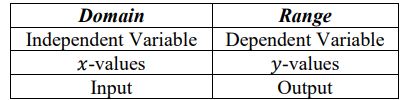• Instruction includes opportunities to use a graphic organizer to chart and provide specific examples of domain and range as compound inequalities.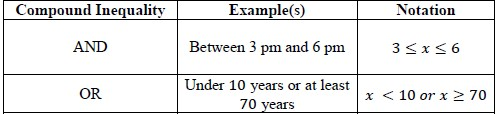• Teacher provides a graphic organizer for each of the three forms of linear equations (standard, slope-intercept and point-slope form) that can be co-created to highlight key similarities and differences.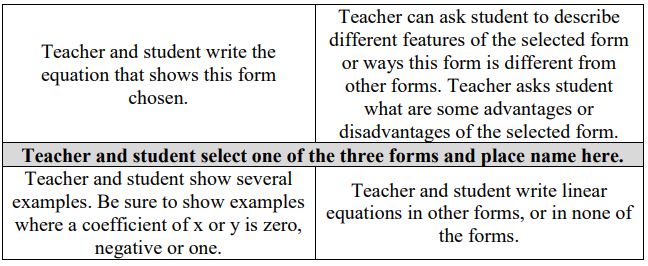• Callie and her friend Elena are reading through different novels they checked out from their school library last Tuesday. Callie’s progress through her novel can be modeled by the function $p$($d$) = −25$d$ + 318, where $p$($d$) represents the number of pages remaining to be read and $d$ is the number of days since receiving the book. Elena’s progress through her novel is modeled by the graph below.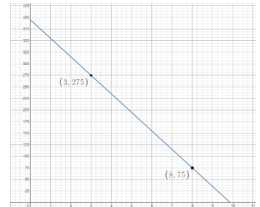• Part A. Which student’s novel has more pages to read?
• Part B. Assuming they both continue to read at a constant rate, which student will complete their novel first?

### Instructional Items

Instructional Item 1
• Two linear functions, $f$($x$) and $g$($x$), are represented below. Compare the functions by stating which has a greater $y$-intercept, $x$-intercept and rate of change.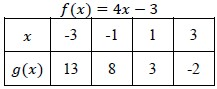*The strategies, tasks and items included in the B1G-M are examples and should not be considered comprehensive.

## Related Courses

This benchmark is part of these courses.
1200310: Algebra 1 (Specifically in versions: 2014 - 2015, 2015 - 2022, 2022 and beyond (current))
1200320: Algebra 1 Honors (Specifically in versions: 2014 - 2015, 2015 - 2022, 2022 and beyond (current))
1200370: Algebra 1-A (Specifically in versions: 2014 - 2015, 2015 - 2022, 2022 and beyond (current))
1200400: Foundational Skills in Mathematics 9-12 (Specifically in versions: 2014 - 2015, 2015 - 2022, 2022 and beyond (current))
7912080: Access Algebra 1A (Specifically in versions: 2014 - 2015, 2015 - 2018, 2018 - 2019, 2019 - 2022, 2022 and beyond (current))
1200315: Algebra 1 for Credit Recovery (Specifically in versions: 2014 - 2015, 2015 - 2022, 2022 and beyond (current))
1200375: Algebra 1-A for Credit Recovery (Specifically in versions: 2014 - 2015, 2015 - 2022, 2022 and beyond (current))
7912075: Access Algebra 1 (Specifically in versions: 2014 - 2015, 2015 - 2018, 2018 - 2019, 2019 - 2022, 2022 and beyond (current))

## Related Access Points

Alternate version of this benchmark for students with significant cognitive disabilities.
MA.912.F.1.AP.5: Identify key features of linear and quadratic functions each represented in the same way algebraically or graphically (key features are limited to domain; range; intercepts; intervals where the function is increasing, decreasing, positive or negative; end behavior).

## Related Resources

Vetted resources educators can use to teach the concepts and skills in this benchmark.

## Formative Assessment

Comparing Linear Functions:

Students are given two linear functions, one represented by a graph and the other by an equation, and asked to compare their intercepts in the context of a problem.

Type: Formative Assessment

## Lesson Plans

Stop That Arguing:

Students will explore representing the movement of objects and the relationship between the various forms of representation: verbal descriptions, value tables, graphs, and equations. These representations include speed, starting position, and direction. This exploration includes brief direct instruction, guided practice in the form of a game, and independent practice in the form of a word problem. Students will demonstrate understanding of this concept through a written commitment of their answer to the word problem supported with evidence from value tables, graphs, and equations.

Type: Lesson Plan

Whose Line Is It Anyway?:

In this lesson, students will use graphing calculators to explore linear equations in the form y = mx + b. They will observe the graphs of equations with different values of slope and y-intercept. They will draw conclusions about how the value of slope and y-intercept are visible in the appearance of the graph.

Type: Lesson Plan

Graphing vs. Substitution. Which would you choose?:

Students will solve multiple systems of equations using two methods: graphing and substitution. This will help students to make a connection between the two methods and realize that they will indeed get the same solution graphically and algebraically.  Students will compare the two methods and think about ways to decide which method to use for a particular problem. This lesson connects prior instruction on solving systems of equations graphically with using algebraic methods to solve systems of equations.

Type: Lesson Plan

My Candles are MELTING!:

In this lesson, students will apply their knowledge to model a real-world linear situation in a variety of ways. They will analyze a situation in which 2 candles burn at different rates. They will create a table of values, determine a linear equation, and graph each to determine if and when the candles will ever be the same height. They will also determine the domain and range of their functions and determine whether there are constraints on their functions.

Type: Lesson Plan

## MFAS Formative Assessments

Comparing Linear Functions:

Students are given two linear functions, one represented by a graph and the other by an equation, and asked to compare their intercepts in the context of a problem.

## Student Resources

Vetted resources students can use to learn the concepts and skills in this benchmark.

## Parent Resources

Vetted resources caregivers can use to help students learn the concepts and skills in this benchmark.